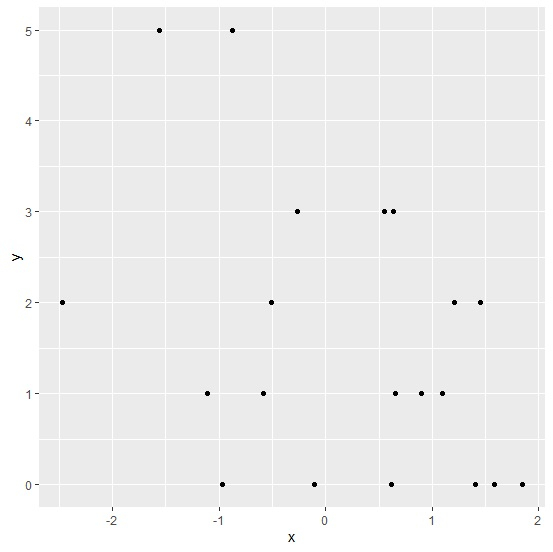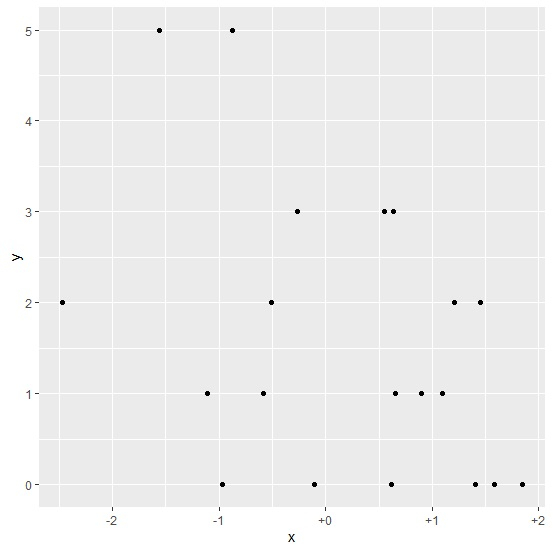# How to display positive sign for X-axis labels in R using ggplot2?

By default, the positive signs are not displayed in any plot in R. It is well known that if there is no sign seen with any value then it is considered positive, therefore, we do not need the sign but to distinguish between 0 and positive values it could be done. To display positive sign for X-axis labels, we can use scale_x_continuous function.

Consider the below data frame −

## Example

Live Demo

x<-rnorm(20)
y<-rpois(20,2)
df<-data.frame(x,y)
df

## Output

      x       y
1 0.6340310   3
2 1.2107246   2
3 -1.1054617  1
4 -0.5074514  2
5 1.4542955   2
6 0.5501350   3
7 -1.5630402  5
8 -0.8708720  5
9 1.8475619    0
10 -0.9714984 0
11 -0.5837147  1
12 -2.4681126  2
13 -0.2683293  3
14 0.9019841   1
15 -0.1082968  0
16 1.5797151   0
17 1.0914647   1
18 1.4080222   0
19 0.6156943   0
20 0.6528113   1

## Example

library(ggplot2)
ggplot(df,aes(x,y))+geom_point()

## OutputCreating scatterplot between x and y having positive labels of X displayed with positive signs −

## Example

ggplot(df,aes(x,y))+geom_point()+scale_x_continuous(labels=function(x) sprintf("%+d",x))

## Output